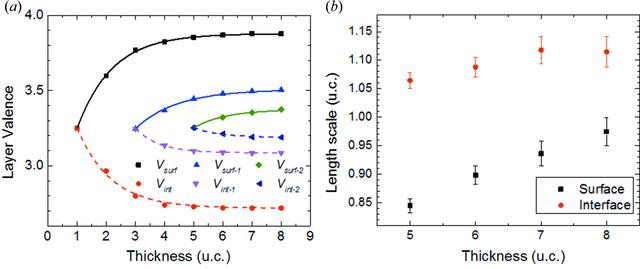disable zoom     view article Figure 7 (a) Variation of the surface and interface-layer valence with thickness as predicted by theory. As each layer follows similar thickness-dependent trends, equations (2)and (3)were employed and the resulting dashed lines are shown. Here Vsurf-n (Vint-n) refers to the valence of the nth layer from the surface (interface). (b) Thickness dependence of the surface and interface-length scales, Ls and Lint, as determined by fitting the theoretical data in Fig. 4to equation (1). Points below 5 u.c. have been omitted as there are a sufficient number of parameters to fit equation (1)exactly to the data, i.e. the error is zero.JOURNAL OFSYNCHROTRONRADIATION
ISSN: 1600-5775
Volume 25| Part 6| November 2018| Pages 1711-1718